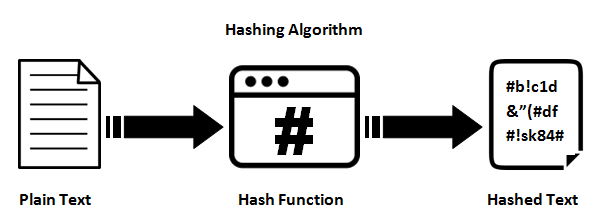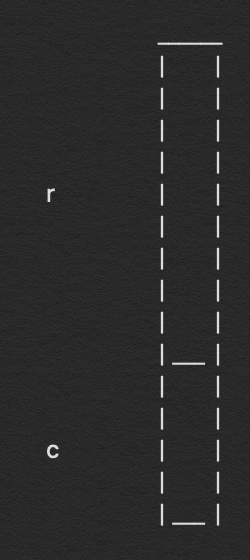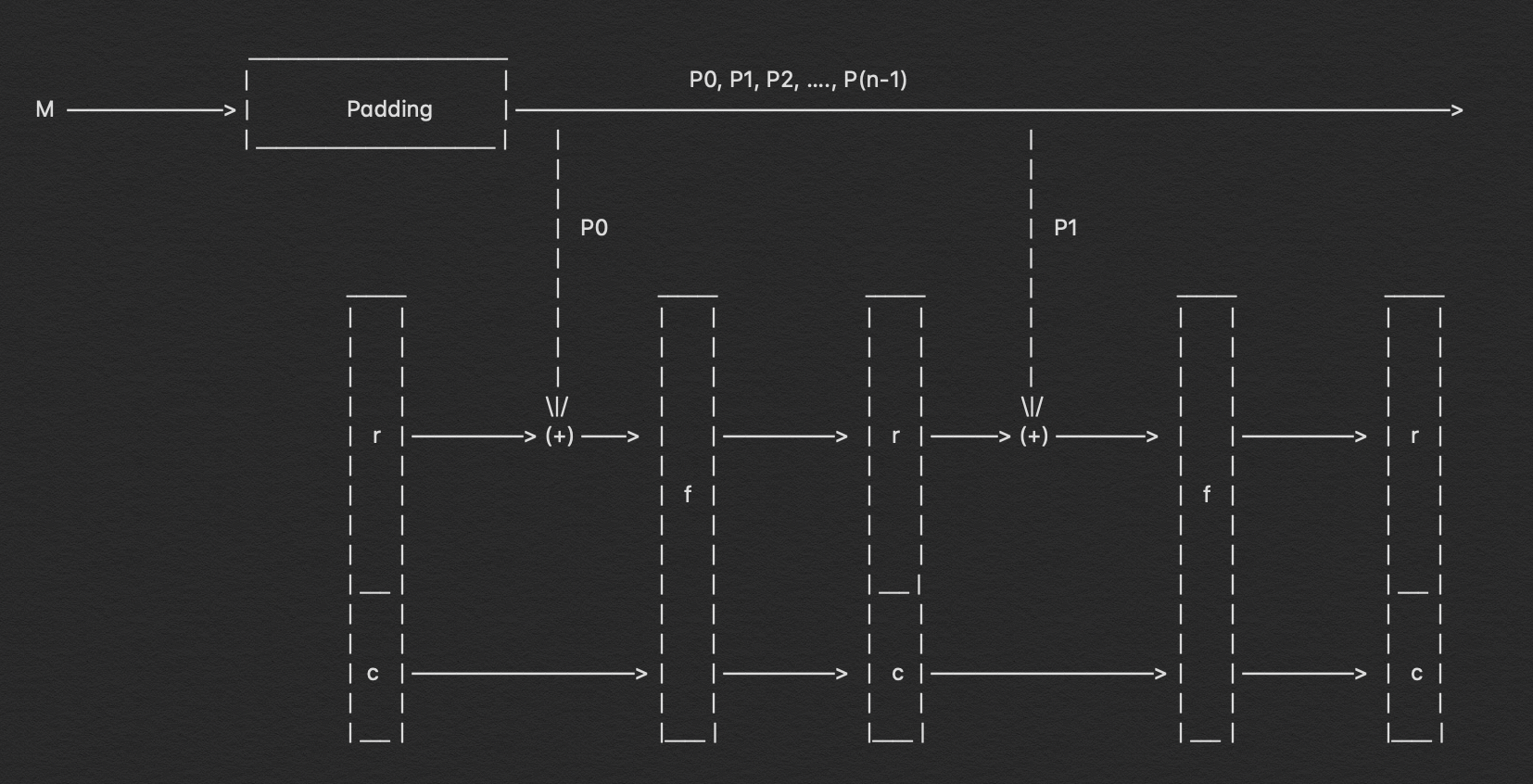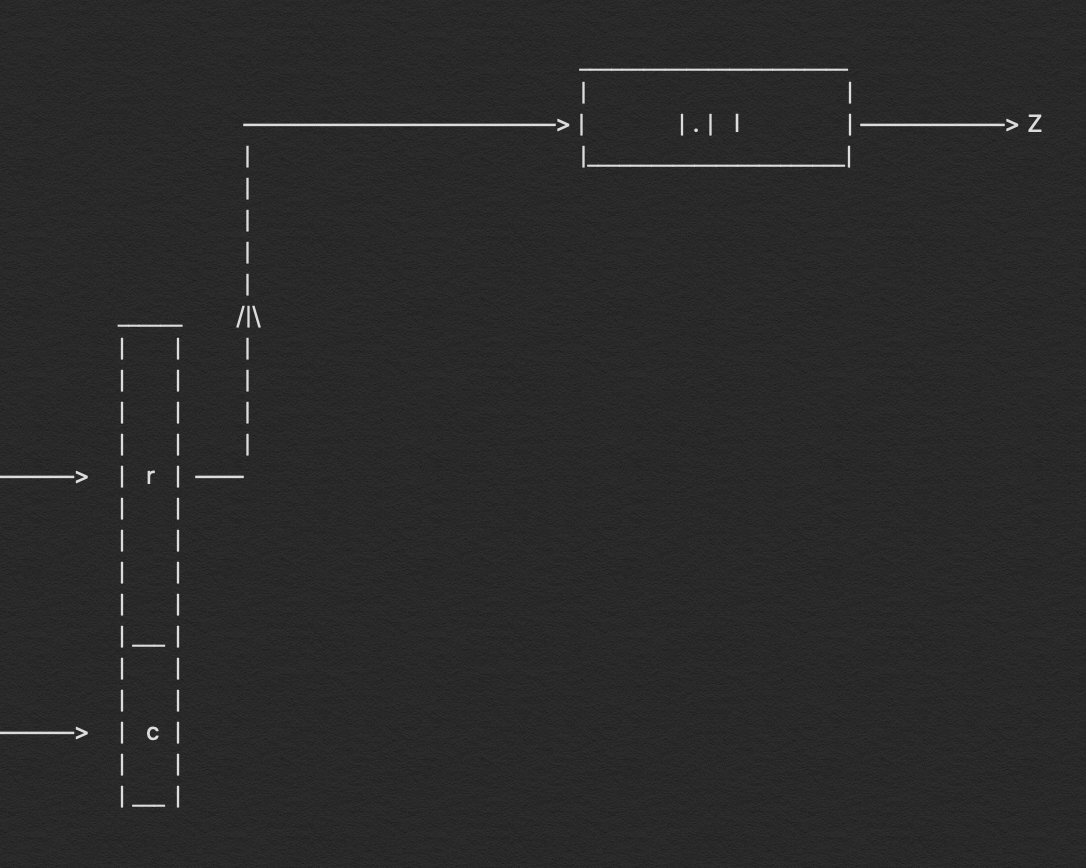Search
•Aditya Anand

# Breaking Down : SHA-3 Algorithm

#### Looking under the hood and understanding how it works?I have been writing about hashing algorithm the whole past month and this is close to the series “Breaking Down”. There are a lot of prominent hashing algorithms that are present in our current day scenario. Especially the ones that came up and competed against each other for the SHA-3 title. So, to give you all a better perspective about the latest and greatest hashing algorithm SHA-3, let me go back a bit in time and explain to you why it came into existence.### History

Around the beginning of 21ˢᵗ century, the SHA-2 algorithm came into existence and the SHA-1 algorithm was already being challenged theoretically. It was evident that it would not hold for a long period of time before collisions would be easy to find. So, in midst of all this NIST started a competition to find a better successor to SHA-2, even though it was secure and it still is.

The competition to find the best and most efficient hash algorithm began and out of 64 submissions, [ * 51 according to Wikipedia ]. Keccak was the one which passed all the rounds and was selected at last to be the SHA-3 algorithm in the year 2015. It was designed by Guido Bertoni, Joan Daemen, Michaël Peeters, and Gilles Van Assche. You should read about its history and how it came into existence, it is interesting.

### Let’s begin!

So, those of you who don’t read my articles on a regular basis, this article is a continuation of my series, where I describe about hashing algorithms and how they function is extreme detail. You can read my other articles, I have mentioned them below.

Breaking Down : The series

1. Breaking Down : MD5 Algorithm

2. Breaking Down: SHA-1 Algorithm

3. Breaking Down : SHA-512 Algorithm

4. Breaking Down : SHA-256 Algorithm

So, now that we know why there was a need for SHA-3 and all about its history. Let's see how it works.

To begin with I need to state that Keccak is not at all similar to the previous algorithms so we need to have a look at it with an open mind or else it might prove a bit hard to understand. Scroll up and have a look at the image at the beginning of the article that will help us have an understanding of the flow of the entire algorithm and how it works.

This is the process that is similar to the previous hashing algorithms. Before we can start hashing our message we need to make sure that they are of the standard length and for that we carry out the padding process.

Before we proceed with it, we need to know what is the standard size we need to meet and for that, we will look at how Keccak calculates the state size.

`b = 25 x 2ˡ ; b = state size`
```value of l = {0, 1, 2, 3, 4, 5, 6}
value of b = {25, 50, 100, 200, 400, 800, 1600}```

For SHA-3 the value of ‘l’ was decided to be 6. Higher the state size better the security it provides. Now, based on the value of ‘l’ we also decide how many rounds of computation needs to be carried out for each part of the padded message.

```rounds = 12 + 2 x l
= 12 + 12    ; as l = 6
= 24         ; 24 rounds in total```

Now, we know that for SHA-3 we will have the state size of 1600 bits and the number of rounds of computations will be 24.

Coming back to padding we need to append bits to message depending on the hash length we are going to calculate. The values should be a multiple of the numbers that I will mention below. For now just remember these values as I explain about them later on.

``` Type        Output Length     Rate (r)         Capacity (c)
SHA3-224           224            1152              448
SHA3-256           256            1088              512
SHA3-384           384             832              768
SHA3-512           512             576             1024```

The padding needs to be done in such a way that after the padding process the length of the padded message is an exact multiple of ‘r’ for the corresponding hash function.

First and the last bit of the padding will be ‘1’ and all bits in between will be ‘0’. After padding they are divided into ‘n’ parts such as n times r is equal to the length of the padded message. Mathematically it can be represented as such.

`p = n x r`
```p = length of message after padding
n = number of parts in which we divide 'p'
r = length of the rate```
Note : The sum of the values of ‘r’ and ‘c’ will always be equal to 1600 i.e. the sate size

#### 2. The state sizeWe now know that the length of the padded message is an exact multiple of ‘r’ for corresponding hash length but to further understand it have a look at the image on your left. The ‘r’ and ‘c’ in the image represent the rate and capacity of the respective hashing algorithm.

As the padded message is an exact multiple of ‘r’ and we need to carry out a modulo operation. So, the length of ‘r’ and P₀ are same.

State size is the sum of ‘r’ and ‘c’ and they have distinct values for different hash length.

#### 3. The Absorb FunctionThe SHA-3 algorithm can be broadly divided into two different parts, the absorbing part and the squeezing part. The absorb function is the first part of the two major steps of the SHA-3 function.

The reason we call it the absorb function is that in the first part of the Keccak algorithm we intake all the values of the padded message that we have already broken down into ‘n’ number of parts and consume them one by one to give the output at the end.

The way in which we perform this, is we feed the ‘r’ length padded messages in the absorbing function. We begin with the modulo operation between P₀ and the ‘r’, the initiation value of ‘r’ is all ‘0’ bits. Once the modulo operation is done then we pass on the value to the function where the actual absorb function begins.

Inside the function, we perform the same set of five operations over and over for twenty-four times. Once all the rounds are over then we segregate the ‘r’ and ‘c’ bits and then again perform the modulo operation and the function begins all over again.

Let’s have a look at the pseudo-code of the five functions:-

θ (theta) : Pseudo-Code

```for x in 0…4
C[x] = A[x,0] xor A[x,1] xor A[x,2] xor A[x,3] xor A[x,4],    ```
```for x in 0…4
D[x] = C[x-1] xor rot(C[x+1],1), ```
```for (x,y) in (0…4,0…4)
A[x,y] = A[x,y] xor D[x]```

ρ (rho) & π (pi) : Pseudo-Code

```for (x,y) in (0…4,0…4)
B[y,2*x+3*y] = rot(A[x,y], r[x,y]),```

χ (chi) : Pseudo-Code

```for (x,y) in (0…4,0…4)
A[x,y] = B[x,y] xor ((not B[x+1,y]) and B[x+2,y])```

ι (iota) : Pseudo-Code

`A[0,0] = A[0,0] xor RC`

It would be hard for me to explain these functions in words, so I presented the pseudo-code from the website of the Keccak team. Read their papers to have a better understanding of the entire concept.

These five functions are carried out over and over for 24 times. After 24 rounds of computation are over we get 1600 bits, which we then segregate depending on the length of ‘r’ and ‘c’ bits and the process continues.

#### 4. The Squeeze Function

The squeeze function begins immediately after we reach the end of the absorb function. We call it the squeeze function as this is the step in which we extract our hash message. The way in which we extract it is extremely simple and easy to understand.When calculating the hash we already know the output length of the hash value which might be 224, 256, 384 or 512. After completing the absorb function we get a final 1600 bits length output. We segregate the output on the basis on length of ‘r’ and ‘c’ bits depending on the hash value we are trying to calculate, which leads us to our output.

### Output

Now, that we have the values for ‘r’ and ‘c’ we then extract the first few bits from ‘r’ depending on the hashing algorithm, so for SHA3–256 algorithm we will extract the first 256 bits from the 1088 bits of ‘r’ and for SHA3–512 we will extract the first 512 bits from the 576 bits of ‘r’. The value that is extracted from the first bits of ‘r’ is the hash of the entire message.

### Conclusion

The SHA-3 / Keccak algorithm is one of the most secure and efficient hashing algorithms and some claim that it won’t be cracked in the next 20 - 30 years. Developments in the quantum computing world might decrease that time frame but it is still one of the best hashing algorithm we have got right now.

So, let’s have a second look at the entire functioning of the SHA-3 algorithm and allow me to explain the entire thing in a single long paragraph.

Our first step as always is to calculate the length of the message and then do the padding process, depending on the hash length we choose to go with. The padding bits that we append to the message start and end with ‘1’ and all the bits in between are ‘0’. Once the padding is completed then we break it up into ‘n’ number of blocks each of ‘r’ length, the value ‘r’ will again depend on the hash length. The padded bits start with P₀ then P₁ till Pₙ-₁. We begin with P₀ for which we first carry out the modulo operation with ‘r’ which initially is all ‘0’. Once, the modulo operation is complete we then begin the 24 rounds each round consists of these five functions θ, ρ, π, χ and ι. and after all these rounds we have the next 1600 bits which we then segregate into ‘r’ and ‘c’ bits depending on the hash length. This computation of 24 rounds takes place ‘n’ number of times in the absorb function and then we reach the squeeze function. From the very beginning, we know the length of the output while carrying out the hash and so we extract those exact number of bits from ‘r’ and that is our complete hash value.

So that’s the short version of the entire operation that takes place in the SHA-3 algorithm.

If you enjoyed it please do clap & let’s collaborate. Get, Set, Hack!